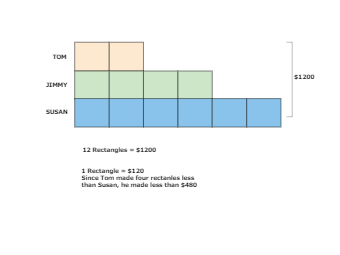Template Community / Tape diagram math example

# Tape diagram math exampleeasy diagrams
Published on 2020-09-16
Edit OnlineA tape diagram is a diagram commonly used to solve comparison mathematical problems. A tape diagram is a rectangular visual model that looks like a piece of tape and is used to help in ratio calculations. A divided bar model, fraction strip, length model, or strip diagram are some other names for it. It is used in mathematics education to solve problems. A tape diagram is a rectangular visual model that looks like a piece of tape and is used to help in ratio calculations. A divided bar model, fraction strip, length model, or strip diagram are some other names for it. It is used to solve word problems in mathematics. issues with words.
Tag
tape diagram
math
Report
0
200PostRecommended Templates